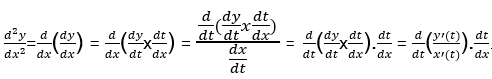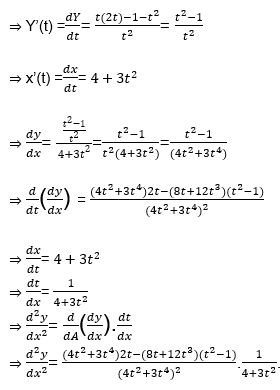# Higher Order Derivatives of Functions in Parametric Form

Sometimes relationships between two variables are represented by using third variable to make things easier to handle. In mathematics this third variable is called a parameter. Mostly, curves are represented by the parameters, for example, x = a and y = 2at. For these types of the curves we have to find the derivative as given below:

$\frac{dy}{dx} = \frac{dy}{dt} \times \frac{dt}{dx} = \frac{y’(t)}{x’(t)}$

In this section, we will solve problems of curves which are defined using parameters, and explain how their derivatives can be found using parametric differentiation.

## Higher-order Derivative in Parametric Form

We know that derivative of the first order in parametric equations is given by:

$\frac{dy}{dx} = \frac{dy}{dt} \times \frac{dt}{ dx} = \frac{y’(t)}{x’(t)}$

Where t is the parameter.

Then if we differentiate the given first derivative w.r.t. x we will get 2nd order derivative as given below:## Solved Examples

Below are few solved examples on first order derivative and higher order derivatives.

### Examples on first order derivative

Problem 1: Find $\frac{dy}{dx}$ if y = sin t and x = cos t.

Solution: y = sin t and x = cos t, then

y’(t) = cos t

x’(t) = – sin t

$\frac{dy}{dx}=\frac{cos(t)}{-sin(t)}$

or $\frac{dy}{dx} = – cot \ t$

Problem 2: Find $\frac{dy}{dx}$ if y = sec3 A and x = a + tan3 A.

Solution: y = sec3 A and x = a + tan3 A, then

⇒ y’(A) = 3 sec2 A.sec A.tan A

And x’(A) = 3 tan2 A . sec2 A

$\frac{dy}{dx}$ = $\frac{3 sec^2 A . sec A . tan A}{3 tan^2 A.sec^2 A}$

$\frac{dy}{dx}$ = $\frac{sec A}{tan A}$

$\frac{dy}{dx}$ = $\frac{1}{sin A}$

$\frac{dy}{dx}$ = cosec A.

### Examples on higher order derivative

Problem 3: Find the 2nd order derivative if x = p(A – sin A) and y = p(1 – cos A)

Solution: x = p(A – sin A) and y = p(1 – cos A) then

⇒ y’(A) = dy/dA = p sin A

⇒ x’(A) = dx/dA = p(1-cos A)

⇒ dy/dx = $\frac{p sin A}{p(1-cos A)}$

⇒ dy/dx = $\frac{2 sin \frac{A}{2} cos \frac{A}{2}}{2sin^2 \frac{A}{2}}$

⇒ dy/dx = $\frac{cos \frac{A}{2}}{sin^2 \frac{A}{2}}$

⇒ dy/dx = $cot \frac{A}{2}$

Now,

$\frac{d}{dA}(\frac{dy}{dx})= \frac{1}{2} \times -cosec^2 (\frac{A}{2})$

and, dx/dA= p(1-cos A)

⇒ dA/dx= $\frac{1}{p(1-cos A)}$

$\frac{d^2 y}{dx^2} = \frac{d}{dA}(\frac{dy}{dx}). \frac{dA}{dx}$

$\frac{d^2 y}{dx^2}= \frac{1}{2} \times -cosec^2 (\frac{A}{2}) \times \frac{1}{(p(1-cos A)}$.

Problem 4: Find the second order derivative of the Y w..r.t. X, Y = $\frac{1+t^2}{t}$= and x = 4t + t3.

Solution: Y = $\frac{1+t^2}{t}$ and x = 4t + t3, then